# Formula's Summary - Sequence and Series Notes | Study Business Mathematics and Logical Reasoning & Statistics - CA Foundation

## CA Foundation: Formula's Summary - Sequence and Series Notes | Study Business Mathematics and Logical Reasoning & Statistics - CA Foundation

The document Formula's Summary - Sequence and Series Notes | Study Business Mathematics and Logical Reasoning & Statistics - CA Foundation is a part of the CA Foundation Course Business Mathematics and Logical Reasoning & Statistics.
All you need of CA Foundation at this link: CA Foundation

1. The general term of an A. P.

a, a + d, a + 2d, ... is given by tn = a + (n –1) d

2. Sn, the sum of the first n terms of an A. P.

a, a + d, a + 2d, ...   is given by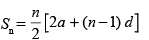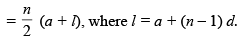3. A sequence in which the difference of two cousecutive terms is always constant ( ≠ 0) is called an Arithmetic Progression (A. P.)

tn = Sn – Sn – 1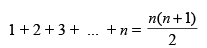4. An arithmetic mean between a and b is (a + b)/2

5. A sequence in which the ratio of two consecutive terms is always constant ( ≠ 0) is called a Geometric Progression (G. P.)

6. The nth term of a G.P.: a, ar, ar2, ... is arn – 1

7. Sum of the first n terms of a G. P.: a, ar, ar2, ... is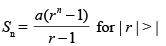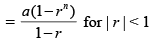8. The sums of an infintite G. P. a, ar, ar2, ... is given by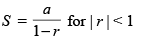9. Geometric mean G between two numbers a and b is √ab

10. The arithmetic mean A between two numbers a and b is always greater than the corresponding Geometric mean G  i.e., A > G.

The document Formula's Summary - Sequence and Series Notes | Study Business Mathematics and Logical Reasoning & Statistics - CA Foundation is a part of the CA Foundation Course Business Mathematics and Logical Reasoning & Statistics.
All you need of CA Foundation at this link: CA FoundationUse Code STAYHOME200 and get INR 200 additional OFF

## Business Mathematics and Logical Reasoning & Statistics

81 videos|101 docs|87 tests

Track your progress, build streaks, highlight & save important lessons and more!

,

,

,

,

,

,

,

,

,

,

,

,

,

,

,

,

,

,

,

,

,

;